# GUIregress

GUIregress shows the necessary calculations to obtain simple linear regression statistics in a GUI.

## Syntax

• out=GUIregress(x,y)example
• out=GUIregress(x,y,Name,Value)example

## Description

 out =GUIregress(x, y) Calculation of unweighted regression.

 out =GUIregress(x, y, Name, Value) Use of option plots.

## Examples

expand all

###Calculation of unweighted regression.

In this example we know the monthly income of 13 families and we estimate the correlation with the free time expenditure. (See page 223 of [MRZ]).

% x= monthly income of 13 families.
% y= free time expenditure.
x=[1330 1225 1225 1400 1575 2050 1750 2240 1225 1730 1470 2730 1380];
y=[120 60 30 60 90 150 140 210 30 100 30 270 260];
out=GUIregress(x,y);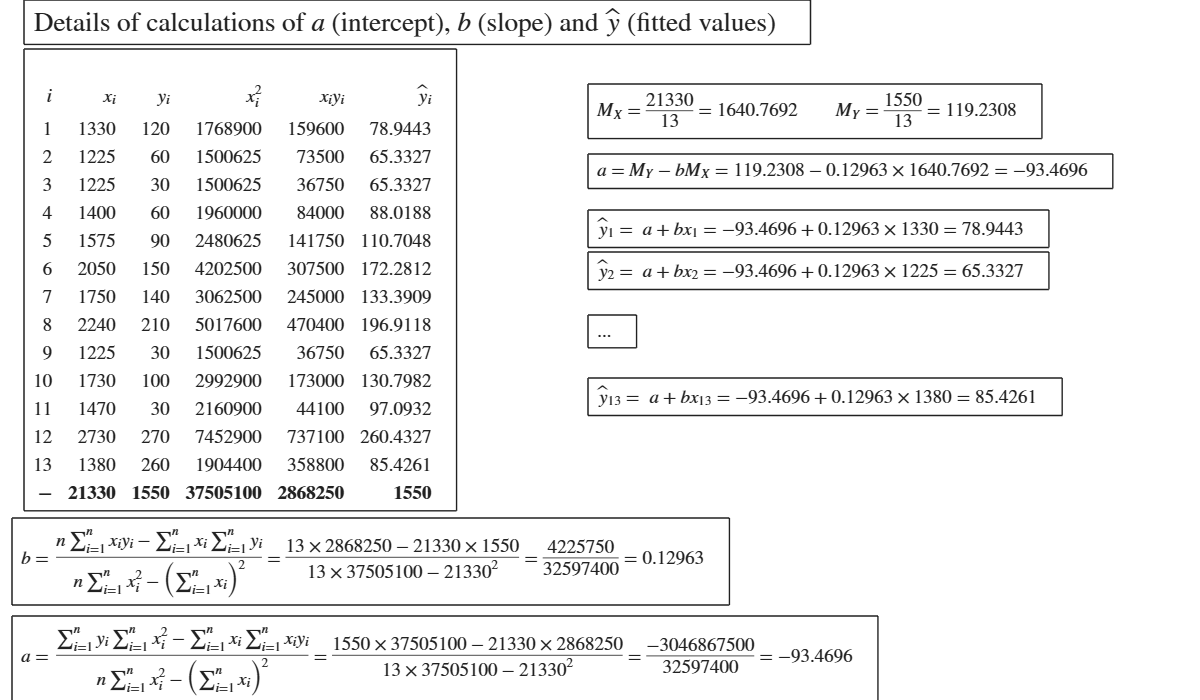###Use of option plots.

The following data matrix reports, for 6 countries, the tourism revenues (y) recorded in a given year (in billions of dollars) and the number of foreign visitors (x) in the same year (in millions of units). (See page 101 of [CMR])

x=[60 48 45 30 23 15];
y=[27.3 25.1 58.4 27.1 17.5 11.9];
out=GUIregress(x,y,'plots',true);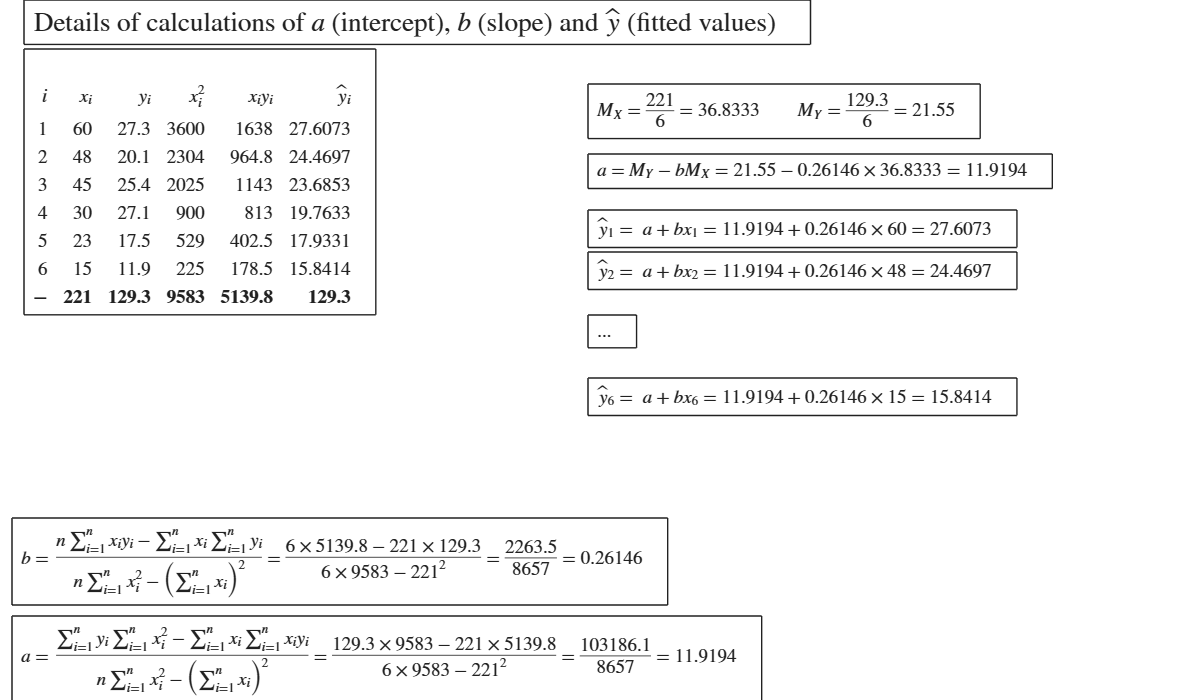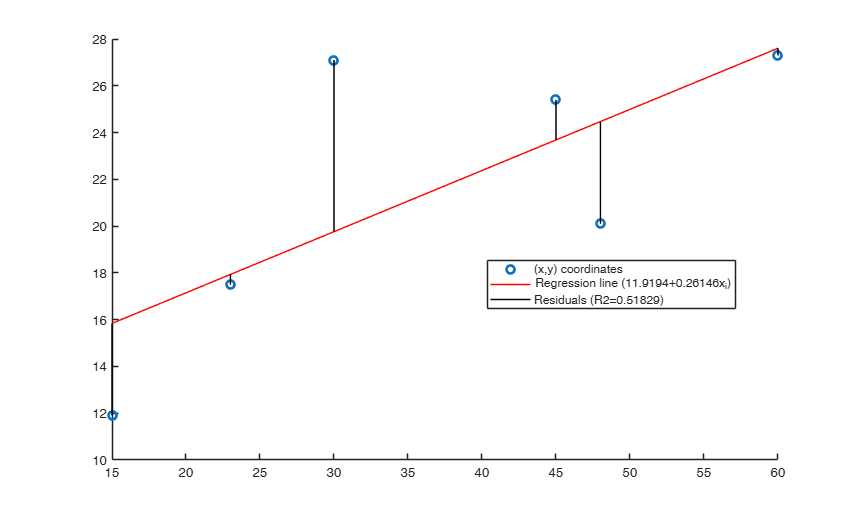## Related Examples

expand all

###Use of option intercept.

The following data matrix reports, for 6 countries, the tourism revenues (y) recorded in a given year (in billions of dollars) and the number of foreign visitors (x) in the same year (in millions of units). (See page 101 of [CMR])

x=[60 48 45 30 23 15];
y=[27.3 25.1 58.4 27.1 17.5 11.9];
out=GUIregress(x,y,'intercept',false);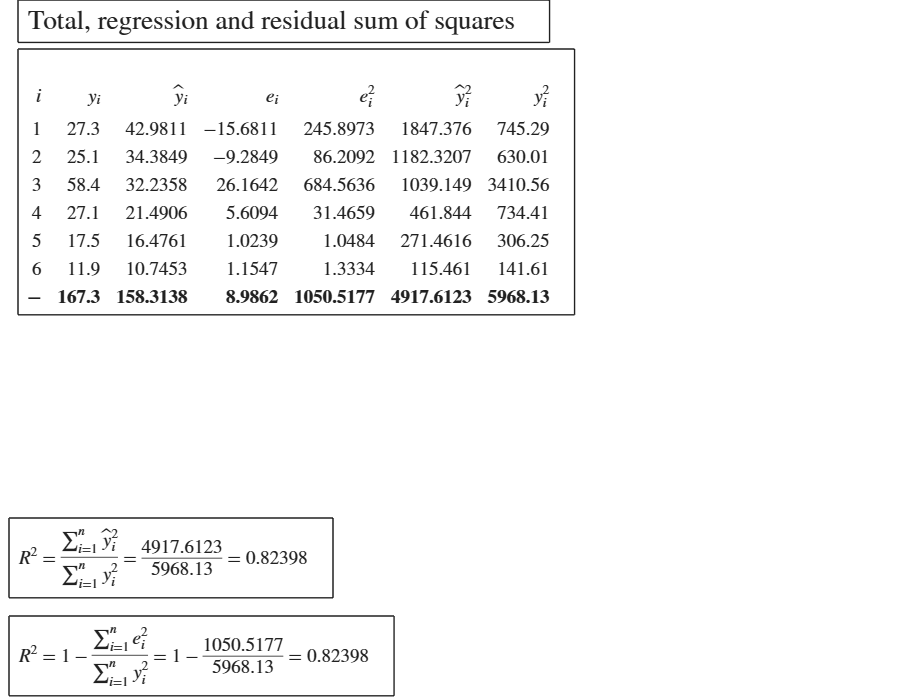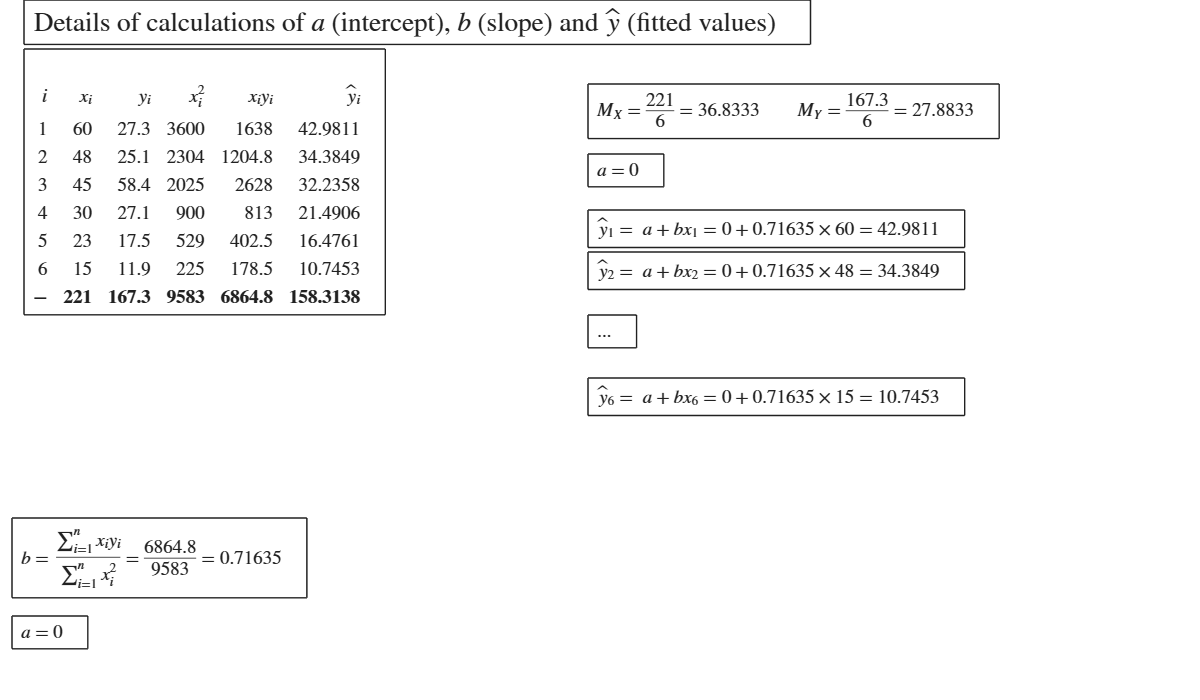### First argument passed as table.

The following data matrix reports, for 6 countries, the tourism revenues (y) recorded in a given year (in billions of dollars) and the number of foreign visitors (x) in the same year (in millions of units). (See page 101 of [CMR])

x=[60 48 45 30 23 15];
y=[27.3 25.1 58.4 27.1 17.5 11.9];
XX=array2table([x' y'],'VariableNames',{'x','y'});
out=GUIregress(XX);

###Example of exponential interpolation.

The values ​​of a company's production, in millions of euros were as follows: (See page 116 of [CMR])

y=[50 52 56 59 65 70 76];
% Analyze the trend of the company's production using an exponential fit.
out=GUIregress([],y,'interpolant','exponential','plots',true);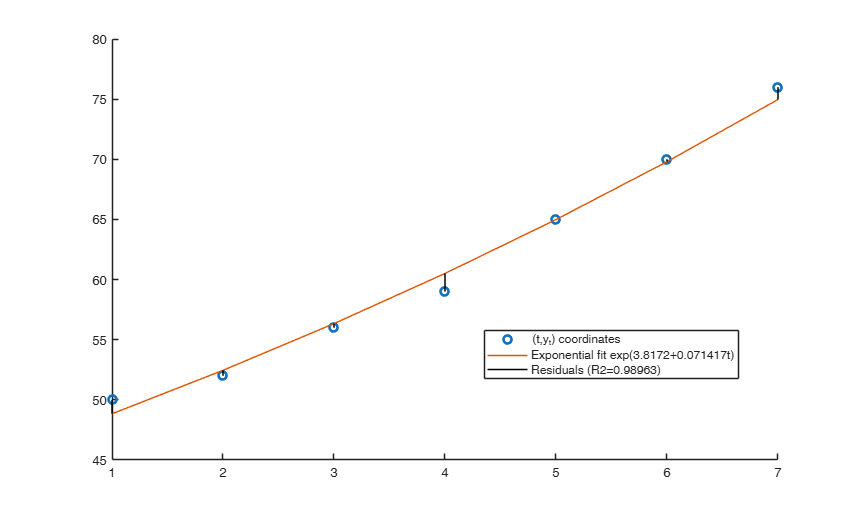###Example of power interpolation.

The values ​​of a company's production, in millions of euros were as follows: (See page 116 of [CMR])

y=[50 52 56 59 65 70 76];
% Analyze the trend of the company's production using a power fit.
out=GUIregress([],y,'interpolant','power','plots',true);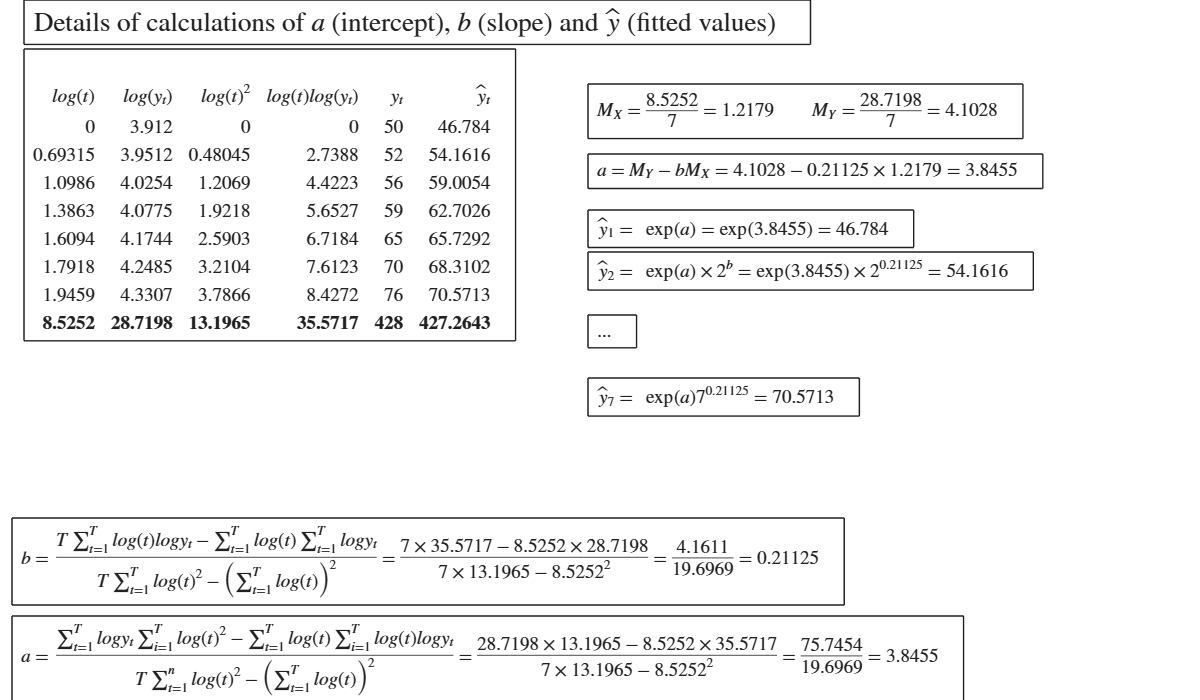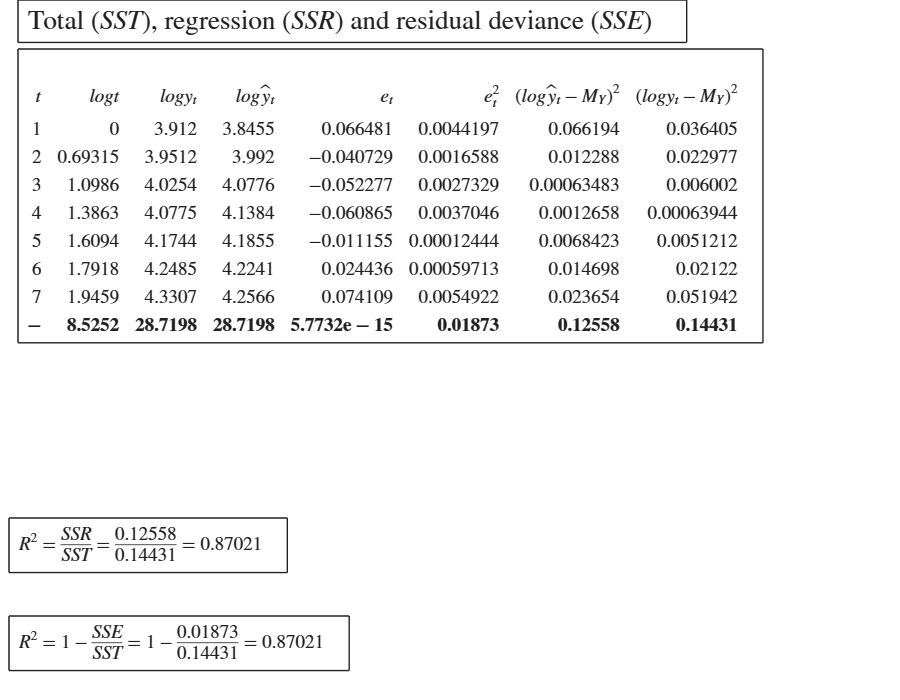## Input Arguments

### x — vector of numeric data or table. Vector or table or empty.

Vector containing strictly numerical data.

If x is table the second input argument y is not necessary. In this case the response is the last column of the table.

If x is empty it is assumed that regression is against time.

Data Types: double or table or []

### y — vector of numeric data. Vector containing strictly numerical data.

This input argument is not requested if previous input argument x is a table.

Data Types: double

### Name-Value Pair Arguments

Specify optional comma-separated pairs of Name,Value arguments. Name is the argument name and Value is the corresponding value. Name must appear inside single quotes (' '). You can specify several name and value pair arguments in any order as  Name1,Value1,...,NameN,ValueN.

Example:  'intercept',false , 'plots',true , 'interpolant','exponential' 

### intercept —Indicator for constant term.true (default) | false.

Indicator for the constant term (intercept) in the fit, specified as the comma-separated pair consisting of 'Intercept' and either true to include or false to remove the constant term from the regression model.

Example:  'intercept',false 

Data Types: boolean

### plots —show regression graphically.boolean.

If plots is true an additional plot which shows the (x,y) data, the fitted regression line and the residuals is shown on the screen. Clicking on the line in the legend it is possible to show/hide these three components.

Example:  'plots',true 

Data Types: boolean

### interpolant —interpolation method.'character'.

Possible values are 'linear' (default) for linear interpolation, 'exponential' for exponential interpolation and 'power' for power interpolation.

Example:  'interpolant','exponential' 

Data Types: char

## Output Arguments

### out —detailed output to compute the index. Table

Table with n+1 rows (where n is the length of x) containing what is shown in the GUI. Last row contains the totals.

Milioli, M.A., Riani, M., Zani, S. (2019), "Introduzione all'analisi dei dati statistici (Quarta edizione ampliata)". [MRZ]

Cerioli, A., Milioli, M.A., Riani, M. (2016), "Esercizi di statistica (Quinta edizione)". [CMR]